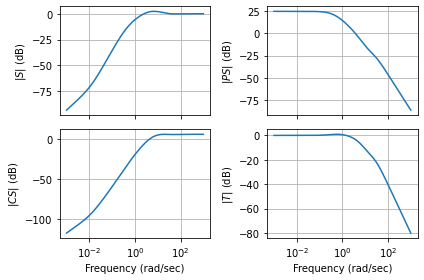# Vertical takeoff and landing aircraft¶

This notebook demonstrates the use of the python-control package for analysis and design of a controller for a vectored thrust aircraft model that is used as a running example through the text Feedback Systems by Astrom and Murray. This example makes use of MATLAB compatible commands.

Additional information on this system is available at

http://www.cds.caltech.edu/~murray/wiki/index.php/Python-control/Example:_Vertical_takeoff_and_landing_aircraft

## System Description¶

This example uses a simplified model for a (planar) vertical takeoff and landing aircraft (PVTOL), as shown below:The position and orientation of the center of mass of the aircraft is denoted by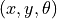,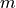is the mass of the vehicle,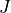the moment of inertia,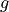the gravitational constant and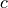the damping coefficient. The forces generated by the main downward thruster and the maneuvering thrusters are modeled as a pair of forces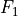and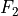acting at a distance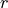below the aircraft (determined by the geometry of the thrusters).

Letting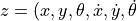), the equations can be written in state space form as: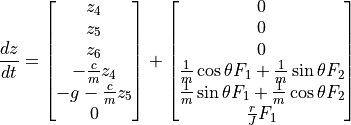## LQR state feedback controller¶

This section demonstrates the design of an LQR state feedback controller for the vectored thrust aircraft example. This example is pulled from Chapter 6 (Linear Systems, Example 6.4) and Chapter 7 (State Feedback, Example 7.9) of Astrom and Murray. The python code listed here are contained the the file pvtol-lqr.py.

To execute this example, we first import the libraries for SciPy, MATLAB plotting and the python-control package:

:

from numpy import *             # Grab all of the NumPy functions
from matplotlib.pyplot import * # Grab MATLAB plotting functions
from control.matlab import *    # MATLAB-like functions
%matplotlib inline


The parameters for the system are given by

:

m = 4                         # mass of aircraft
J = 0.0475                    # inertia around pitch axis
r = 0.25                      # distance to center of force
g = 9.8                       # gravitational constant
c = 0.05                      # damping factor (estimated)


Choosing equilibrium inputs to be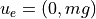, the dynamics of the system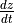, and their linearization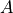about equilibrium point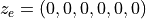are given by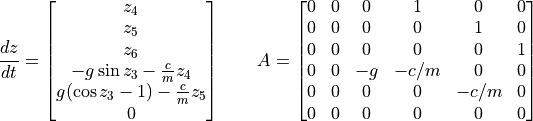:

# State space dynamics
xe = [0, 0, 0, 0, 0, 0]         # equilibrium point of interest
ue = [0, m*g]                   # (note these are lists, not matrices)

:

# Dynamics matrix (use matrix type so that * works for multiplication)
# Note that we write A and B here in full generality in case we want
# to test different xe and ue.
A = matrix(
[[ 0,    0,    0,    1,    0,    0],
[ 0,    0,    0,    0,    1,    0],
[ 0,    0,    0,    0,    0,    1],
[ 0, 0, (-ue*sin(xe) - ue*cos(xe))/m, -c/m, 0, 0],
[ 0, 0, (ue*cos(xe) - ue*sin(xe))/m, 0, -c/m, 0],
[ 0,    0,    0,    0,    0,    0 ]])

# Input matrix
B = matrix(
[[0, 0], [0, 0], [0, 0],
[cos(xe)/m, -sin(xe)/m],
[sin(xe)/m,  cos(xe)/m],
[r/J, 0]])

# Output matrix
C = matrix([[1, 0, 0, 0, 0, 0], [0, 1, 0, 0, 0, 0]])
D = matrix([[0, 0], [0, 0]])


To compute a linear quadratic regulator for the system, we write the cost function as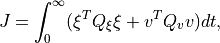where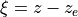and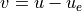represent the local coordinates around the desired equilibrium point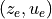. We begin with diagonal matrices for the state and input costs:

:

Qx1 = diag([1, 1, 1, 1, 1, 1])
Qu1a = diag([1, 1])
(K, X, E) = lqr(A, B, Qx1, Qu1a); K1a = matrix(K)


This gives a control law of the form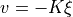, which can then be used to derive the control law in terms of the original variables: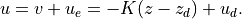whereand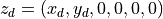The way we setup the dynamics above,is already hardcoding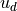, so we don’t need to include it as an external input. So we just need to cascade the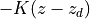controller with the PVTOL aircraft’s dynamics to control it. For didactic purposes, we will cheat in two small ways:

• First, we will only interface our controller with the linearized dynamics. Using the nonlinear dynamics would require the NonlinearIOSystem functionalities, which we leave to another notebook to introduce.

1. Second, as written, our controller requires full state feedback (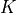multiplies full state vectors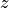), which we do not have access to because our system, as written above, only returns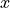and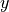(because of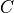matrix). Hence, we would need a state observer, such as a Kalman Filter, to track the state variables. Instead, we assume that we have access to the full state.

The following code implements the closed loop system:

:

# Our input to the system will only be (x_d, y_d), so we need to
# multiply it by this matrix to turn it into z_d.
Xd = matrix([[1,0,0,0,0,0],
[0,1,0,0,0,0]]).T

# Closed loop dynamics
H = ss(A-B*K,B*K*Xd,C,D)

# Step response for the first input
x,t = step(H,input=0,output=0,T=linspace(0,10,100))
# Step response for the second input
y,t = step(H,input=1,output=1,T=linspace(0,10,100))

:

plot(t,x,'-',t,y,'--')
plot([0, 10], [1, 1], 'k-')
ylabel('Position')
xlabel('Time (s)')
title('Step Response for Inputs')
legend(('Yx', 'Yy'), loc='lower right')
show()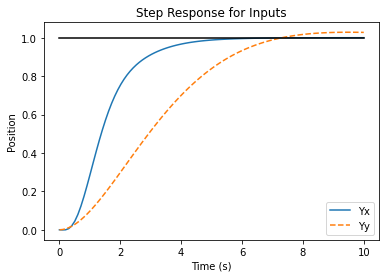The plot above shows theandpositions of the aircraft when it is commanded to move 1 m in each direction. The following shows themotion for control weights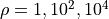. A higher weight of the input term in the cost function causes a more sluggish response. It is created using the code:

:

# Look at different input weightings
Qu1a = diag([1, 1])
K1a, X, E = lqr(A, B, Qx1, Qu1a)
H1ax = H = ss(A-B*K1a,B*K1a*Xd,C,D)

Qu1b = (40**2)*diag([1, 1])
K1b, X, E = lqr(A, B, Qx1, Qu1b)
H1bx = H = ss(A-B*K1b,B*K1b*Xd,C,D)

Qu1c = (200**2)*diag([1, 1])
K1c, X, E = lqr(A, B, Qx1, Qu1c)
H1cx = ss(A-B*K1c,B*K1c*Xd,C,D)

[Y1, T1] = step(H1ax, T=linspace(0,10,100), input=0,output=0)
[Y2, T2] = step(H1bx, T=linspace(0,10,100), input=0,output=0)
[Y3, T3] = step(H1cx, T=linspace(0,10,100), input=0,output=0)

:

plot(T1, Y1.T, 'b-', T2, Y2.T, 'r-', T3, Y3.T, 'g-')
plot([0 ,10], [1, 1], 'k-')
title('Step Response for Inputs')
ylabel('Position')
xlabel('Time (s)')
legend(('Y1','Y2','Y3'),loc='lower right')
axis([0, 10, -0.1, 1.4])
show()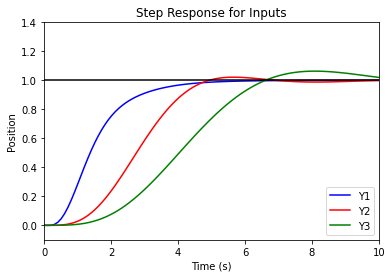## Lateral control using inner/outer loop design¶

This section demonstrates the design of loop shaping controller for the vectored thrust aircraft example. This example is pulled from Chapter 11 (Frequency Domain Design) of Astrom and Murray.

To design a controller for the lateral dynamics of the vectored thrust aircraft, we make use of a “inner/outer” loop design methodology. We begin by representing the dynamics using the block diagramThe controller is constructed by splitting the process dynamics and controller into two components: an inner loop consisting of the roll dynamics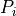and control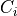and an outer loop consisting of the lateral position dynamics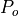and controller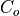.The closed inner loop dynamics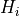control the roll angle of the aircraft using the vectored thrust while the outer loop controllercommands the roll angle to regulate the lateral position.

The following code imports the libraries that are required and defines the dynamics:

:

from matplotlib.pyplot import * # Grab MATLAB plotting functions
from control.matlab import *    # MATLAB-like functions

:

# System parameters
m = 4                         # mass of aircraft
J = 0.0475                    # inertia around pitch axis
r = 0.25                      # distance to center of force
g = 9.8                       # gravitational constant
c = 0.05                      # damping factor (estimated)

:

# Transfer functions for dynamics
Pi = tf([r], [J, 0, 0])       # inner loop (roll)
Po = tf(, [m, c, 0])       # outer loop (position)


For the inner loop, use a lead compensator

:

k = 200
a = 2
b = 50
Ci = k*tf([1, a], [1, b])     # lead compensator
Li = Pi*Ci


The closed loop dynamics of the inner loop,, are given by

:

Hi = parallel(feedback(Ci, Pi), -m*g*feedback(Ci*Pi, 1))


Finally, we design the lateral compensator using another lead compenstor

:

# Now design the lateral control system
a = 0.02
b = 5
K = 2
Co = -K*tf([1, 0.3], [1, 10])         # another lead compensator
Lo = -m*g*Po*Co


The performance of the system can be characterized using the sensitivity function and the complementary sensitivity function:

:

L = Co*Hi*Po
S = feedback(1, L)
T = feedback(L, 1)

:

t, y = step(T, T=linspace(0,10,100))
plot(y, t)
title("Step Response")
grid()
xlabel("time (s)")
ylabel("y(t)")
show()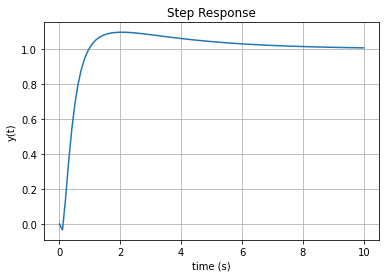The frequency response and Nyquist plot for the loop transfer function are computed using the commands

:

bode(L)
show()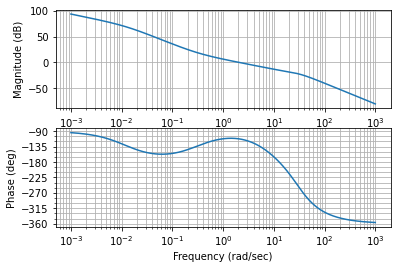:

nyquist(L, (0.0001, 1000))
show()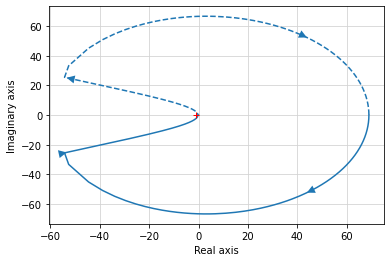:

gangof4(Hi*Po, Co)